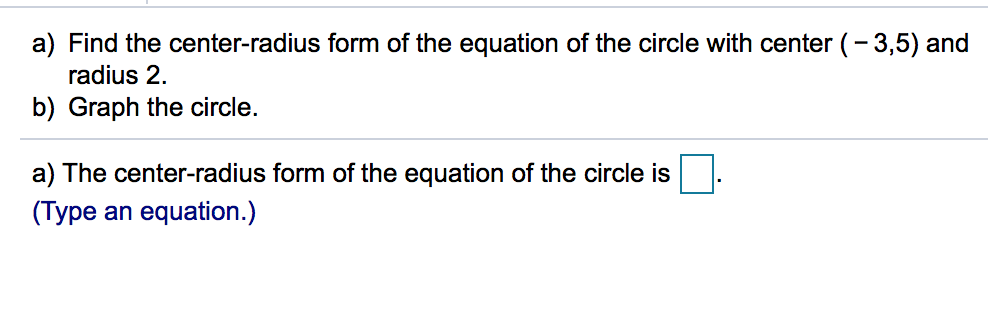# a) Find the center-radius form of the equation of the circle with center (-3,5) and radius 2. b) Graph the circle. a) The center-radius form of the equation of the circle is (Type an equation.)

Questionhelp_outlineImage Transcriptionclosea) Find the center-radius form of the equation of the circle with center (-3,5) and radius 2. b) Graph the circle. a) The center-radius form of the equation of the circle is (Type an equation.) fullscreen

## Expert Answer

### Want to see this answer and more?

Experts are waiting 24/7 to provide step-by-step solutions in as fast as 30 minutes!*

*Response times vary by subject and question complexity. Median response time is 34 minutes and may be longer for new subjects.
Tagged in
MathAlgebra

### Other© 2021 bartleby. All Rights Reserved.